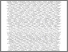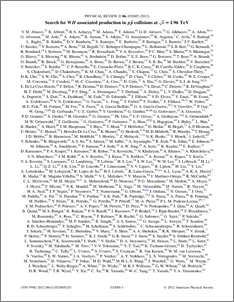# Search for $WH$ associated production in $p \bar{p}$ collisions at $\sqrt{s}=1.96\,{\rm TeV}$

Collaboration, D0 and Bertram, Iain and Borissov, Guennadi and Fox, Harald and Ross, Anthony and Williams, Mark and Ratoff, Peter (2012) Search for $WH$ associated production in $p \bar{p}$ collisions at $\sqrt{s}=1.96\,{\rm TeV}$. Physical Review D, 86 (3). ISSN 1550-7998Preview
PDF
PhysRevD.86.032005.pdf - Published Version

## Abstract

This report describes a search for associated production of $W$ and Higgs bosons based on data corresponding to an integrated luminosity of $\cal{L}$$\approx 5.3 \rm fb^{-1}$ collected with the D0 detector at the Fermilab Tevatron $p\bar{p}$ Collider. Events containing a $W\rightarrow \ell \nu$ candidate (with $\ell$ corresponding to $e$ or $\mu$) are selected in association with two or three reconstructed jets. One or two of the jets are required to be consistent with having evolved from a $b$ quark. A multivariate discriminant technique is used to improve the separation of signal and backgrounds. Expected and observed upper limits are obtained for the product of the $WH$ production cross section and branching ratios and reported in terms of ratios relative to the prediction of the standard model as a function of the mass of the Higgs boson ($M_{H}$). The observed and expected 95% C.L. upper limits obtained for an assumed $M_{H}=115 \rm GeV$ are, respectively, factors of 4.5 and 4.8 larger than the value predicted by the standard model.

Item Type:
Journal Article
Journal or Publication Title:
Physical Review D